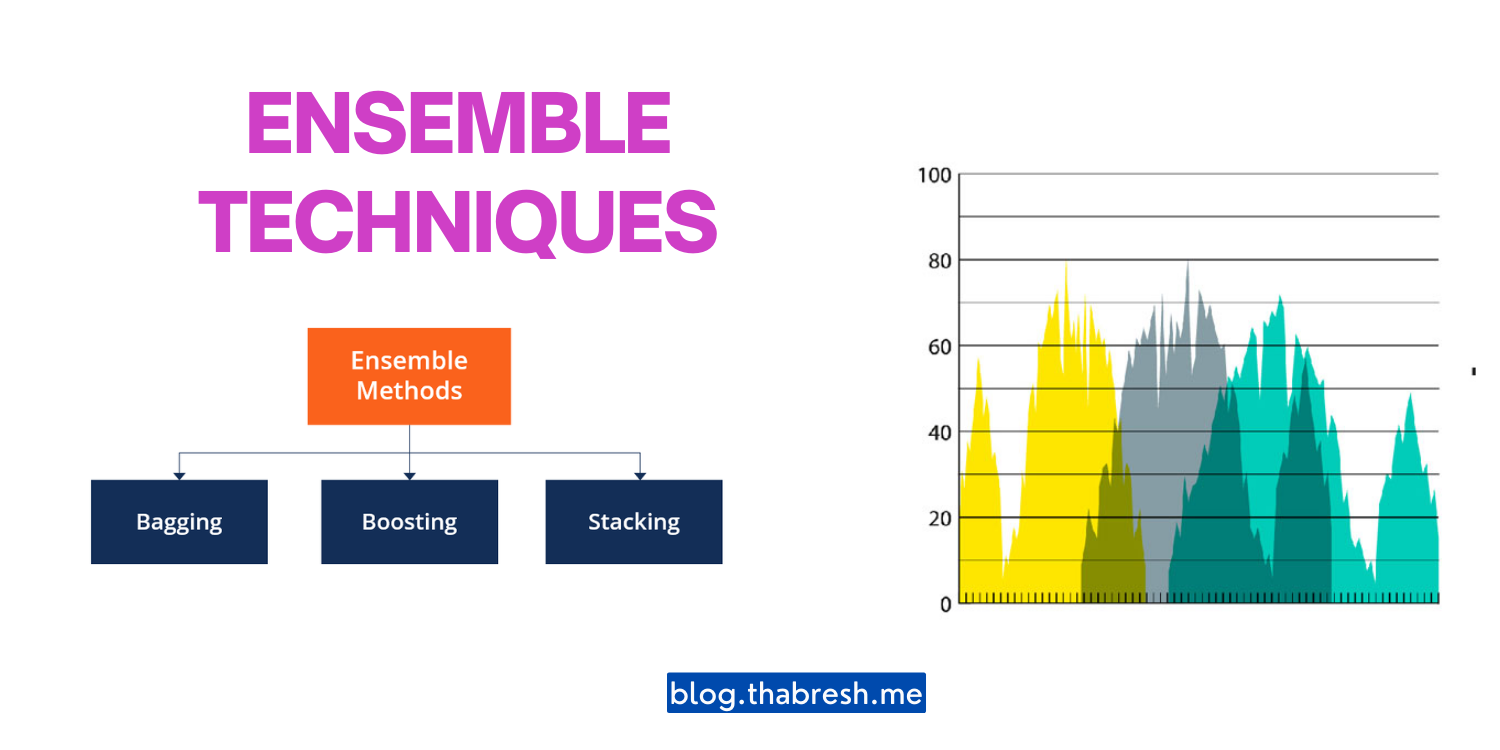Posts

# 📢 Ensemble Techniques: Boosting the Power of Machine Learning Models 🚀## Ensemble Techniques

Ensemble techniques are powerful methods in machine learning that combine multiple models to achieve better performance and accuracy. By leveraging the collective wisdom of multiple models, ensemble techniques have become widely popular and have proven to be highly effective in various domains.

## 🔬 Types of Ensemble Techniques

1️⃣ Bagging

Bagging, short for bootstrap aggregating, involves creating multiple subsets of the training data by sampling with replacement. Each subset is used to train a separate model, and their predictions are combined through averaging or voting to make the final prediction.

2️⃣ Boosting

Boosting involves training a sequence of models, with each subsequent model aiming to correct the mistakes made by the previous models. It assigns weights to the training instances, focusing on the misclassified ones to improve overall performance.

3️⃣ Random Forest

Random Forest is a popular ensemble technique that combines the concept of bagging with decision trees. It creates multiple decision trees by randomly selecting subsets of features and samples from the training data. The predictions of the individual trees are aggregated to make the final prediction.

4️⃣ Stacking

Stacking combines predictions from multiple models by training a meta-model that learns how to best combine the predictions of the base models. The base models are typically diverse in nature, capturing different aspects of the data, and their outputs are used as features for the meta-model.

AdaBoost is a boosting technique that assigns weights to training instances and trains a sequence of models. It adjusts the weights based on the performance of each model, allowing subsequent models to focus more on misclassified instances.

## 📝 Code Example (Random Forest in Python using scikit-learn)

```from sklearn.ensemble import RandomForestClassifier
from sklearn.model_selection import train_test_split
from sklearn.metrics import accuracy_score

X, y = iris.data, iris.target

# Split the data into training and test sets
X_train, X_test, y_train, y_test = train_test_split(X, y, test_size=0.2, random_state=42)

# Create a Random Forest classifier
rf_classifier = RandomForestClassifier(n_estimators=100)

# Train the classifier
rf_classifier.fit(X_train, y_train)

# Make predictions on the test set
y_pred = rf_classifier.predict(X_test)

# Calculate accuracy
accuracy = accuracy_score(y_test, y_pred)
print("Accuracy:", accuracy)

```

In this code example, we use the scikit-learn library to create a Random Forest classifier. The Iris dataset is loaded, split into training and test sets, and the classifier is trained using the training data. Predictions are made on the test set, and the accuracy of the model is calculated.

Ensemble techniques offer a powerful approach to improve the performance and robustness of machine learning models. By combining the predictions of multiple models, they can handle complex patterns and generalize well to unseen data. Experimenting with different ensemble techniques and tuning their parameters can further enhance their effectiveness in solving various machine learning problems. Give them a try and boost your models to new heights! 📈

Related Posts

## Example: Iris Dataset

Thank You So Much for Reading 📢 Ensemble Techniques: Boosting the Power of Machine Learning Models 🚀 Article.